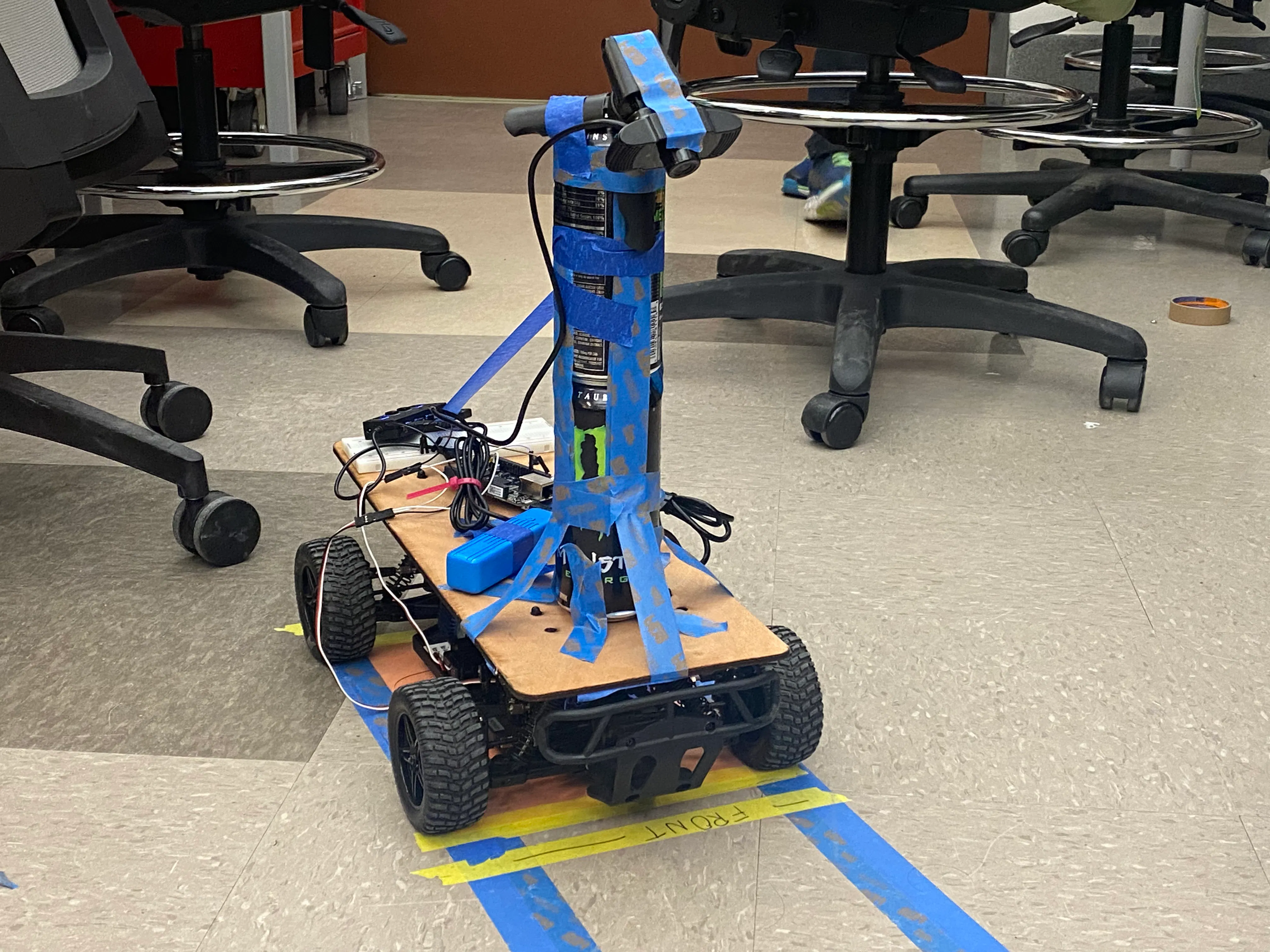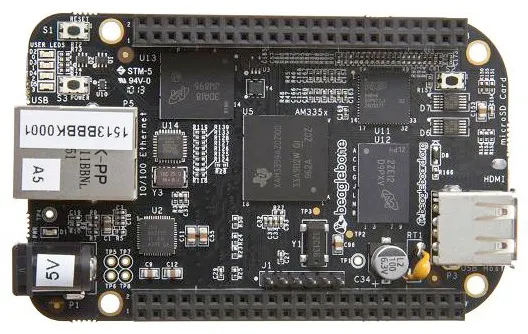Published

# Autonomous RC Car

Our system is designed to use computer vision to have an RC car follow a particular path and stop when it detects the color red.

IntermediateFull instructions provided649## Things used in this project

### Hardware componentsBeagleBoard.org BeagleBone Black
×1
×1
 Webcam
×1
 USB Hub
×1
 Monster Energy
×2

### Software apps and online servicesOpenCV

## Code

### main.py

Python
The main lane detection code that calls on the PID code
```#User raja_961, Autonomous Lane-Keeping Car Using Raspberry Pi and OpenCV. Instructables.
#URL: https://www.instructables.com/Autonomous-Lane-Keeping-Car-Using-Raspberry-Pi-and/
import cv2
import numpy as np
import math
import time
import simple_pid
import Adafruit_BBIO.PWM as PWM
from matplotlib import pyplot as plt

prev_frame = 0
new_frame = 0

def convert_to_HSV(frame):
hsv = cv2.cvtColor(frame, cv2.COLOR_BGR2HSV)
cv2.imshow("HSV",hsv)
return hsv

def detect_edges(frame):
lower_blue = np.array([90, 50, 0], dtype = "uint8") # lower limit of blue color
upper_blue = np.array([120, 255, 255], dtype="uint8") # upper limit of blue color
mask = cv2.inRange(hsv,lower_blue,upper_blue) # this mask will filter out everything but blue

# detect edges
edges = cv2.Canny(mask, 60, 100)
cv2.imshow("edges",edges)
return edges

def detect_stop(frame):
stop = 0
#detect red if it fits in lower and higher thresholds of read color on both ends of the color spectrum
lower_red = np.array([0,50,50])
upper_red = np.array([10,255,255])
mask0 = cv2.inRange(hsv, lower_red, upper_red)
lower_red = np.array([170,50,50])
upper_red = np.array([180,255,255])
mask1 = cv2.inRange(hsv, lower_red, upper_red)
#if frame has at least 100 pixels of red then there must be a stop sign
if hasStop > 100:
stop = 1
print("Stop sign detected!")
return stop

def region_of_interest(edges):
height, width = edges.shape # extract the height and width of the edges frame
mask = np.zeros_like(edges) # make an empty matrix with same dimensions of the edges frame

# only focus lower half of the screen
# specify the coordinates of 4 points (lower left, upper left, upper right, lower right)
polygon = np.array([[
(0, height*(8/8)),
(0,  height*(5/8)),
(width , height*(5/8)),
(width , height*(8/8)),
]], np.int32)

cv2.fillPoly(mask, polygon, 255) # fill the polygon with blue color
cropped_edges = cv2.bitwise_and(edges, mask)
cv2.imshow("roi",cropped_edges)
return cropped_edges

def detect_line_segments(cropped_edges):
rho = 1
theta = np.pi / 180
min_threshold = 10
line_segments = cv2.HoughLinesP(cropped_edges, rho, theta, min_threshold,
np.array([]), minLineLength=2, maxLineGap=1)
return line_segments

def average_slope_intercept(frame, line_segments):
lane_lines = []

if line_segments is None:
print("no line segment detected")
return lane_lines

height, width,_ = frame.shape
left_fit = []
right_fit = []
boundary = 1/3

left_region_boundary = width * (1 - boundary)
right_region_boundary = width * boundary

for line_segment in line_segments:
for x1, y1, x2, y2 in line_segment:
if x1 == x2:
print("skipping vertical lines (slope = infinity)")
continue

fit = np.polyfit((x1, x2), (y1, y2), 1)
slope = (y2 - y1) / (x2 - x1)
intercept = y1 - (slope * x1)

if slope < 0:
if x1 < left_region_boundary and x2 < left_region_boundary:
left_fit.append((slope, intercept))
else:
if x1 > right_region_boundary and x2 > right_region_boundary:
right_fit.append((slope, intercept))

left_fit_average = np.average(left_fit, axis=0)
if len(left_fit) > 0:
lane_lines.append(make_points(frame, left_fit_average))

right_fit_average = np.average(right_fit, axis=0)
if len(right_fit) > 0:
lane_lines.append(make_points(frame, right_fit_average))

# lane_lines is a 2-D array consisting the coordinates of the right and left lane lines
# for example: lane_lines = [[x1,y1,x2,y2],[x1,y1,x2,y2]]
# where the left array is for left lane and the right array is for right lane
# all coordinate points are in pixels
return lane_lines

def make_points(frame, line):
height, width, _ = frame.shape
slope, intercept = line
y1 = height  # bottom of the frame
y2 = int(y1 / 2)  # make points from middle of the frame down

if slope == 0:
slope = 0.1

x1 = int((y1 - intercept) / slope)
x2 = int((y2 - intercept) / slope)

return [[x1, y1, x2, y2]]

def display_lines(frame, lines, line_color=(0, 255, 0), line_width=6): # line color (B,G,R)
line_image = np.zeros_like(frame)

if lines is not None:
for line in lines:
for x1, y1, x2, y2 in line:
cv2.line(line_image, (x1, y1), (x2, y2), line_color, line_width)

line_image = cv2.addWeighted(frame, 0.8, line_image, 1, 1)
return line_image

def get_steering_angle(frame, lane_lines):
height, width, _ = frame.shape

if len(lane_lines) == 2: # if two lane lines are detected
_, _, left_x2, _ = lane_lines # extract left x2 from lane_lines array
_, _, right_x2, _ = lane_lines # extract right x2 from lane_lines array
mid = int(width / 2)
x_offset = (left_x2 + right_x2) / 2 - mid
y_offset = int(height / 2)

elif len(lane_lines) == 1: # if only one line is detected
x1, _, x2, _ = lane_lines
x_offset = (x2 - x1)*.7
y_offset = int(height / 2)

elif len(lane_lines) == 0: # if no line is detected
x_offset = 0
y_offset = int(height / 2)

angle_to_mid_radian = math.atan(x_offset / y_offset)
angle_to_mid_deg = int(angle_to_mid_radian * 180.0 / math.pi)
steering_angle = angle_to_mid_deg + 90

return steering_angle

def display_heading_line(frame, steering_angle, line_color=(0, 0, 255), line_width=5 ):

height, width, _ = frame.shape

steering_angle_radian = steering_angle / 180.0 * math.pi
x1 = int(width / 2)
y1 = height
x2 = int(x1 - height / 2 / math.tan(steering_angle_radian))
y2 = int(height / 2)

cv2.line(heading_image, (x1, y1), (x2, y2), line_color, line_width)

video = cv2.VideoCapture(0)
#initialize states
state = [0,0]
#initialize parameters
params = [.25,.2,0]
speedPWM = 7.5
PWM.start("P9_14",7.5, 50, 0)

#intialize variables
i = 0
check = 1
dont_check = 0
stop_num = 0

#create lists for plotting
speedPWM_list = []
steerPWM_list = []
error_list = []
der_resp_list = []
prop_resp_list = []

# The loop
while True:
#motor only starts after 50 frames of video read
if i > 50:
speedPWM = 7.915
PWM.start("P9_14",7.915, 50,0) #7.87
#set resolution to 100x60 for each frame
frame = cv2.resize(frame,(100,60),fx=0,fy=0, interpolation = cv2.INTER_CUBIC)
#convert frame to hsv
hsv = convert_to_HSV(frame)
edges = detect_edges(hsv)
#if check = 1, check whether there is a stop sign
if check == 1:
stop = detect_stop(hsv)
#if stop sign recently seen, (last 50 frames) then dont check for a stop sign
else:
#count 50 frames before allowing for stop sign check
if dont_check != 50:
dont_check += 1
else:
dont_check = 0
check = 1
if stop == 1:
#count the number of stop signs seen, stop the car if stop sign seen
stop_num += 1
speedPWM = 7.5
PWM.start("P9_14", 7.5, 50, 0)
check = 0
#if second stop sign seen then car stops permanently
if stop_num == 2:
break
#car stops for 2 seconds at first stop sign
time.sleep(2)
#car speeds up again
speedPWM = 7.915
PWM.start("P9_14", 7.915, 50, 0)
stop = 0
#functions for getting steering angle from from blue line detection
roi = region_of_interest(edges)
line_segments = detect_line_segments(roi)
lane_lines = average_slope_intercept(frame,line_segments)
lane_lines_image = display_lines(frame,lane_lines)
steering_angle = (get_steering_angle(frame, lane_lines))
#input steering angle into pid python file to and return steering PWM, derivative response, and proportional response
steerPWM,der_resp,prop_resp = simple_pid.update_steer(state,-(steering_angle-90),params,5)
#plot lane lines and steering angle
i += 1
#add values into their respective lists
speedPWM_list.append(speedPWM)
steerPWM_list.append(steerPWM)
prop_resp_list.append(prop_resp)
der_resp_list.append(der_resp)
error_list.append(steering_angle - 90)
key = cv2.waitKey(1)
if key == 27:
break
#reset PWM values to rest
PWM.start("P9_14", 7.5,50,0)
PWM.start("P8_13", 7.5,50,0)

video.release()
cv2.destroyAllWindows()

#plots
plt.plot(error_list,label = "Error")
plt.plot(speedPWM_list, label = "Speed PWM")
plt.plot(steerPWM_list, label = "Steer PWM")

plt.title("Error and PWM")
plt.legend(loc = 'best')
plt.savefig('ErrorAndPWM.png')

plt.clf()

plt.plot(error_list,label = "Error")
plt.plot(prop_resp_list, label= "Proportional Response")
plt.plot(der_resp_list, label = "Derivative Response")

plt.title("Error and Response")
plt.legend(loc = 'best')
plt.savefig('ErrorAndResponse.png')
```

### Simple_Pid

Python
PID control used in main file
```import Adafruit_BBIO.PWM as PWM
import numpy as np
import math

def update_steer(state,angle,params,v): #updates steering control
steer = "P8_13" #pin asssinment for steering servo
d = angle-state #calculates discrete time derivative of error
state = angle #saves current error in state variable for next update
state = state+angle #caululates discrete time integral of error
action = (params*angle)+(params*d)+(params*state)+0.000001 #calculates PID response
steerpwm = w_to_command(action,v) #getspwm value of desired angular velocity
PWM.start(steer, steerpwm, 50, 0) #commands steering
return(steerpwm,(d*params),params*angle) #returns values of interest

def w_to_command(w,v): #converts angular velocity and velocity to steering PWM
intan = 11/((v*180)/(w*math.pi))
action =  ((np.arctan(intan)*(180/math.pi))+97.055)/13.055 #fitting function
if action>9: #clamps output to steering range
return 9
if action<6:
return 6
return action
```

## Credits

### Tyler Montague

1 project • 0 followers

### Tremayne Currie

1 project • 0 followers

### Jakob Valdez

1 project • 0 followers

### Kj Dix

1 project • 0 followers